Subsections

# Applications of Ising Model

## Ising Model and Lattice Gas

Ising model was invented to describe phase transition in magnetics. It also describes gas-liquid phase transition!

Consider a lattice model: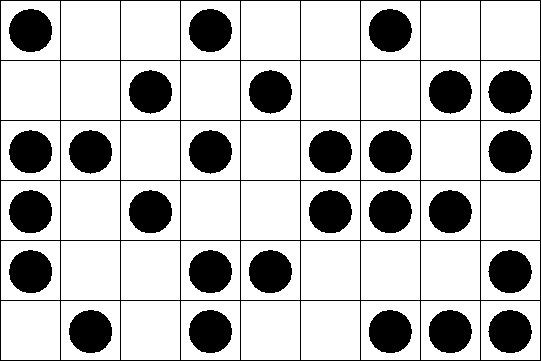Each site can be either occupied or empty. Only nearest neighbors interact. Energy of interaction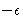. We want to map this to Ising model. Introduce a spin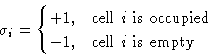Number of particles in a cell: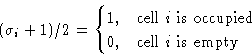Total number of particles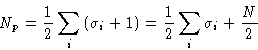Interaction energy between cells 1 and 2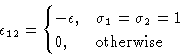This can be written as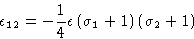Total energy: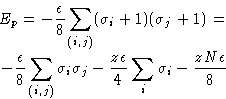Grand partition function: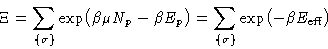(2)
with effective energy: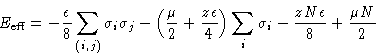This is (up to a constant) the Hamiltonian of an Ising system with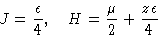Correspondence between Ising magnetic & lattice gas (up to a constant or a factor):
 Ising Magnetic Lattice Gas Canonical ensemble Grand canonical ensemble Coupling constant J Interaction energy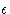External field H Chemical potential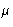Magnetization M Density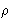Free energy A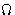-potential; pressure P Susceptibility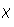Compressibility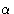Isotropic phase Supercritical fluid Ordered phase Liquid or gas Curie point Critical point

In Ising model phase transition occur at H=0. In lattice gas it corresponds to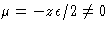--there is no symmetry between particles & holes!

## Ising Model and Lattice Binary Mixture

An incompressible mixture of A and B: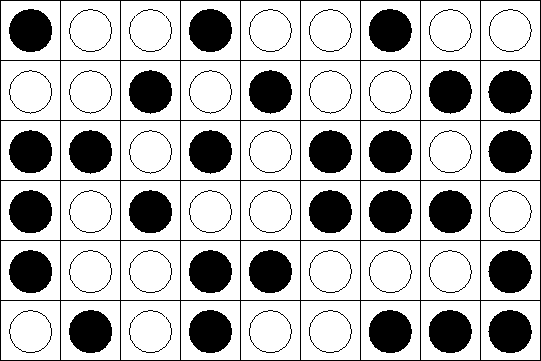Once again, only closest neighbor interact. Energy of interaction: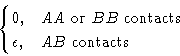We introduce spins: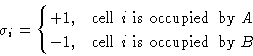Energy of interaction between 1 and 2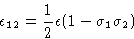Total energy: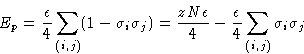Difference between the numbers of particles: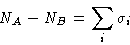I cannot use grand canonical ensemble: the total # of particles is constant (incompressibility). Semi-Grand Ensemble: the total number of particle is constant, but I can switch A and B.

Partition function: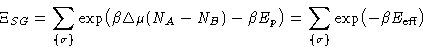with effective energy: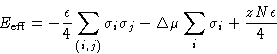Once again Ising model with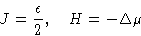Correspondence between Ising magnetic & binary mixture:

 Ising Magnetic Binary Mixture Canonical ensemble Semi-grand canonical ensemble Coupling constant J Interaction energyExternal field H Chemical potential diff.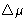Magnetization M Composition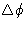Isotropic phase Mixed phase Ordered phase Separated phase Curie point Critical mixing point

## Ising-like Models

How can we extend Ising model?

• Let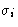have several values (Potts model)--useful for compressible multicomponent mixtures
• Introduce vectorand write interaction as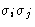(Heisenberg model)
• Introduce complex lattices (fcc, bcc, trigonal, hexagonal...)
• Introduce several sub lattices (decorated models)
• Introduce interaction of farther neighbors and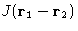• Make J random--favorite toy in glass theory
• Introduce kinetics or re-orientation--make your model time-dependent!Next: Conclusion Up: Ising Model and Its Previous: Phase Transitions of Ising

© 1997 Boris Veytsman and Michael Kotelyanskii
Mon Oct 13 22:07:20 EDT 1997